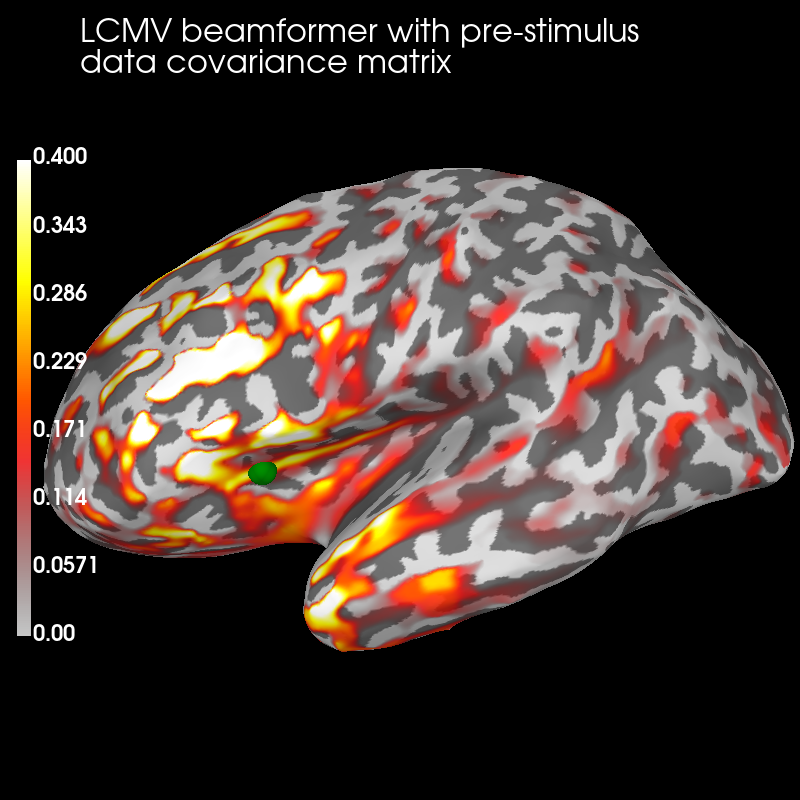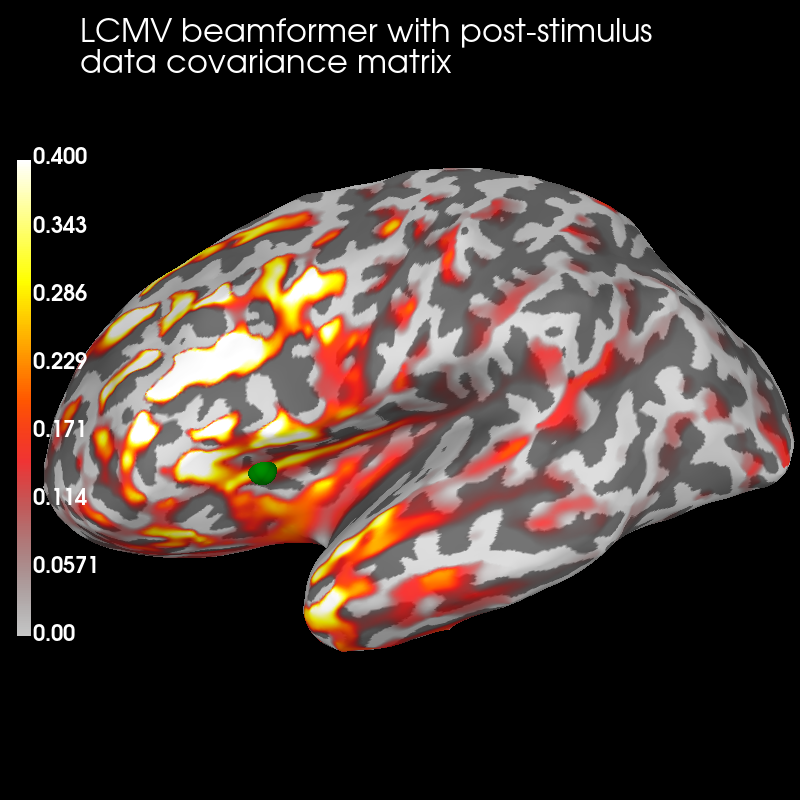# Compute cross-talk functions for LCMV beamformers#

Visualise cross-talk functions at one vertex for LCMV beamformers computed with different data covariance matrices, which affects their cross-talk functions.

```# Author: Olaf Hauk <olaf.hauk@mrc-cbu.cam.ac.uk>
#
```
```import mne
from mne.beamformer import make_lcmv, make_lcmv_resolution_matrix
from mne.datasets import sample
from mne.minimum_norm import get_cross_talk

print(__doc__)

data_path = sample.data_path()
subjects_dir = data_path / "subjects"
meg_path = data_path / "MEG" / "sample"
fname_fwd = meg_path / "sample_audvis-meg-eeg-oct-6-fwd.fif"
fname_cov = meg_path / "sample_audvis-cov.fif"
fname_evo = meg_path / "sample_audvis-ave.fif"
raw_fname = meg_path / "sample_audvis_filt-0-40_raw.fif"

# only pick good EEG/MEG sensors
picks = mne.pick_types(raw.info, meg=True, eeg=True, exclude="bads")

# Find events
events = mne.find_events(raw)

# event_id = {'aud/l': 1, 'aud/r': 2, 'vis/l': 3, 'vis/r': 4}
event_id = {"vis/l": 3, "vis/r": 4}

tmin, tmax = -0.2, 0.25  # epoch duration
epochs = mne.Epochs(
raw,
events,
event_id=event_id,
tmin=tmin,
tmax=tmax,
picks=picks,
baseline=(-0.2, 0.0),
)
del raw

# covariance matrix for pre-stimulus interval
tmin, tmax = -0.2, 0.0
cov_pre = mne.compute_covariance(epochs, tmin=tmin, tmax=tmax, method="empirical")

# covariance matrix for post-stimulus interval (around main evoked responses)
tmin, tmax = 0.05, 0.25
cov_post = mne.compute_covariance(epochs, tmin=tmin, tmax=tmax, method="empirical")
info = epochs.info
del epochs

# use forward operator with fixed source orientations
mne.convert_forward_solution(forward, surf_ori=True, force_fixed=True, copy=False)

# regularize noise covariance (we used 'empirical' above)
noise_cov = mne.cov.regularize(noise_cov, info, mag=0.1, grad=0.1, eeg=0.1, rank="info")
```
```Opening raw data file /home/circleci/mne_data/MNE-sample-data/MEG/sample/sample_audvis_filt-0-40_raw.fif...
Read a total of 4 projection items:
PCA-v1 (1 x 102)  idle
PCA-v2 (1 x 102)  idle
PCA-v3 (1 x 102)  idle
Average EEG reference (1 x 60)  idle
Range : 6450 ... 48149 =     42.956 ...   320.665 secs
319 events found on stim channel STI 014
Event IDs: [ 1  2  3  4  5 32]
143 matching events found
Applying baseline correction (mode: mean)
Created an SSP operator (subspace dimension = 4)
4 projection items activated
Computing rank from data with rank=None
Using tolerance 4.9e-09 (2.2e-16 eps * 305 dim * 7.3e+04  max singular value)
Estimated rank (mag + grad): 302
MEG: rank 302 computed from 305 data channels with 3 projectors
Using tolerance 2.8e-11 (2.2e-16 eps * 59 dim * 2.1e+03  max singular value)
Estimated rank (eeg): 58
EEG: rank 58 computed from 59 data channels with 1 projector
Created an SSP operator (subspace dimension = 4)
Setting small MEG eigenvalues to zero (without PCA)
Setting small EEG eigenvalues to zero (without PCA)
Reducing data rank from 364 -> 360
Estimating covariance using EMPIRICAL
Done.
Number of samples used : 4433
[done]
Computing rank from data with rank=None
Using tolerance 5.9e-09 (2.2e-16 eps * 305 dim * 8.7e+04  max singular value)
Estimated rank (mag + grad): 302
MEG: rank 302 computed from 305 data channels with 3 projectors
Using tolerance 3.8e-11 (2.2e-16 eps * 59 dim * 2.9e+03  max singular value)
Estimated rank (eeg): 58
EEG: rank 58 computed from 59 data channels with 1 projector
Created an SSP operator (subspace dimension = 4)
Setting small MEG eigenvalues to zero (without PCA)
Setting small EEG eigenvalues to zero (without PCA)
Reducing data rank from 364 -> 360
Estimating covariance using EMPIRICAL
Done.
Number of samples used : 4433
[done]
Computing patch statistics...
[done]
Computing patch statistics...
[done]
Desired named matrix (kind = 3523) not available
Read MEG forward solution (7498 sources, 306 channels, free orientations)
Desired named matrix (kind = 3523) not available
Read EEG forward solution (7498 sources, 60 channels, free orientations)
Forward solutions combined: MEG, EEG
Source spaces transformed to the forward solution coordinate frame
Average patch normals will be employed in the rotation to the local surface coordinates....
Converting to surface-based source orientations...
[done]
366 x 366 full covariance (kind = 1) found.
Read a total of 4 projection items:
PCA-v1 (1 x 102) active
PCA-v2 (1 x 102) active
PCA-v3 (1 x 102) active
Average EEG reference (1 x 60) active
Computing rank from covariance with rank='info'
MEG: rank 302 after 3 projectors applied to 305 channels
EEG: rank 58 after 1 projector applied to 59 channels
8 projection items activated
MAG regularization : 0.1
Created an SSP operator (subspace dimension = 3)
Computing rank from covariance with rank={'meg': 302, 'eeg': 58}
Using tolerance 2.5e-14 (2.2e-16 eps * 102 dim * 1.1  max singular value)
Estimated rank (mag): 99
MAG: rank 99 computed from 102 data channels with 3 projectors
Setting small MAG eigenvalues to zero (without PCA)
Computing rank from covariance with rank={'meg': 302, 'eeg': 58, 'mag': 99}
Using tolerance 1.8e-13 (2.2e-16 eps * 203 dim * 3.9  max singular value)
GRAD: rank 203 computed from 203 data channels with 0 projectors
Setting small GRAD eigenvalues to zero (without PCA)
EEG regularization : 0.1
Created an SSP operator (subspace dimension = 1)
Computing rank from covariance with rank={'meg': 302, 'eeg': 58, 'mag': 99, 'grad': 203}
Setting small EEG eigenvalues to zero (without PCA)
```

## Compute LCMV filters with different data covariance matrices#

```# compute LCMV beamformer filters for pre-stimulus interval
filters_pre = make_lcmv(
info,
forward,
cov_pre,
reg=0.05,
noise_cov=noise_cov,
pick_ori=None,
rank=None,
weight_norm=None,
reduce_rank=False,
verbose=False,
)

# compute LCMV beamformer filters for post-stimulus interval
filters_post = make_lcmv(
info,
forward,
cov_post,
reg=0.05,
noise_cov=noise_cov,
pick_ori=None,
rank=None,
weight_norm=None,
reduce_rank=False,
verbose=False,
)
```

## Compute resolution matrices for the two LCMV beamformers#

```# compute cross-talk functions (CTFs) for one target vertex
sources = 
verttrue = [forward["src"]["vertno"][sources]]  # pick one vertex
rm_pre = make_lcmv_resolution_matrix(filters_pre, forward, info)
stc_pre = get_cross_talk(rm_pre, forward["src"], sources, norm=True)
del rm_pre
```
```    364 out of 366 channels remain after picking
Dimensions of LCMV resolution matrix: 7498 by 7498.
```
```rm_post = make_lcmv_resolution_matrix(filters_post, forward, info)
stc_post = get_cross_talk(rm_post, forward["src"], sources, norm=True)
del rm_post
```
```    364 out of 366 channels remain after picking
Dimensions of LCMV resolution matrix: 7498 by 7498.
```

## Visualize#

Pre:

```brain_pre = stc_pre.plot(
"sample",
"inflated",
"lh",
subjects_dir=subjects_dir,
figure=1,
clim=dict(kind="value", lims=(0, 0.2, 0.4)),
)

0.1,
0.9,
"LCMV beamformer with pre-stimulus\ndata " "covariance matrix",
"title",
font_size=16,
)

# mark true source location for CTFs
verttrue, coords_as_verts=True, scale_factor=1.0, hemi="lh", color="green"
)
```Post:

```brain_post = stc_post.plot(
"sample",
"inflated",
"lh",
subjects_dir=subjects_dir,
figure=2,
clim=dict(kind="value", lims=(0, 0.2, 0.4)),
)

0.1,
0.9,
"LCMV beamformer with post-stimulus\ndata " "covariance matrix",
"title",
font_size=16,
)